Question

1. What is the final volume in milliliters when 0.653 L of a 45.4 % (m/v)...

1. What is the final volume in milliliters when 0.653 L of a 45.4 % (m/v) solution is diluted to 24.0 % (m/v)?

2. A 751 mL NaCl solution is diluted to a volume of 1.06 L and a concentration of 8.00 M . What was the initial concentration?

3. What volume of 1.00 M HCl in liters is needed to react completely (with nothing left over) with 0.750 L of 0.200 M Na2CO3?

Express your answer to three significant figures and include the appropriate units.

4. A 639-mL sample of unknown HCl solution reacts completely with Na2CO3 to form 15.1 g CO2. What was the concentration of the HCl solution?

Express your answer to three significant figures and include the appropriate units.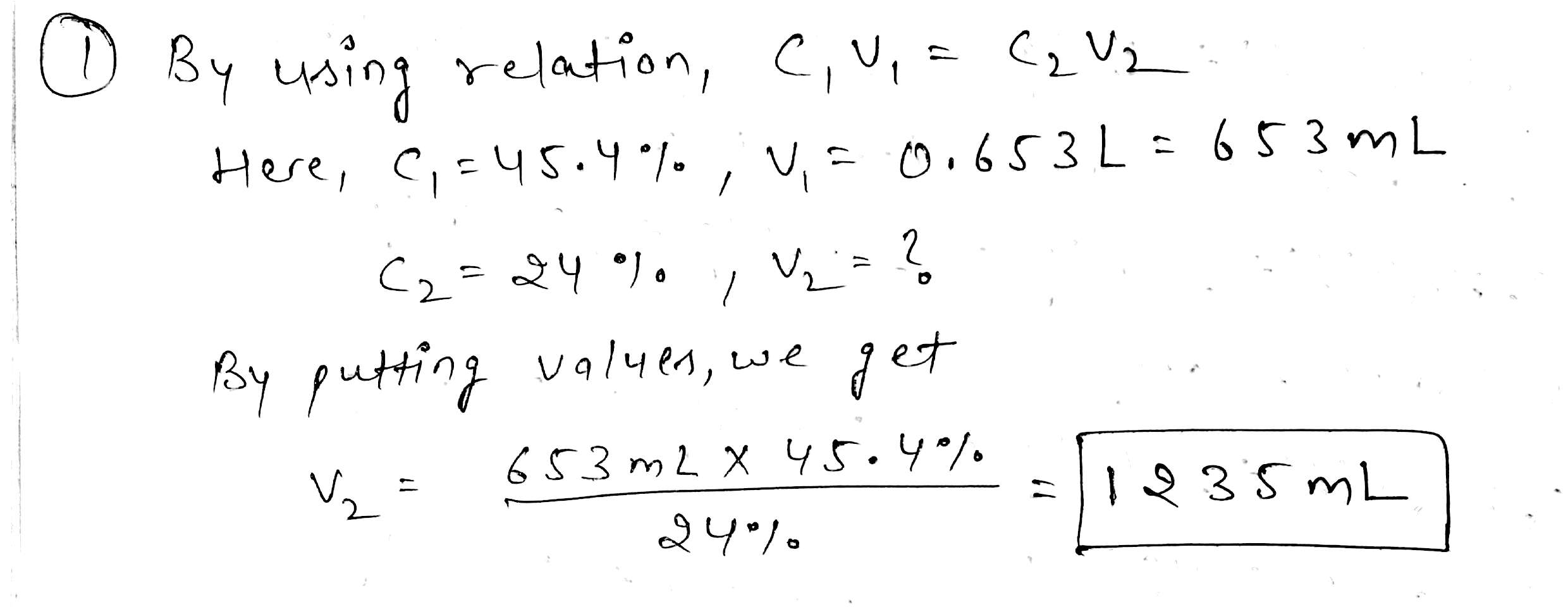Earn Coins

Coins can be redeemed for fabulous gifts.

Similar Homework Help Questions
• 1. What volume of 1.00 M HCl in liters is needed to react completely (with nothing...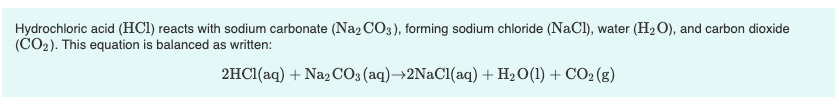1. What volume of 1.00 M HCl in liters is needed to react completely (with nothing left over) with 0.750 L of 0.200 M Na2CO3? Express your answer to three significant figures and include the appropriate units. 2. A 639-mL sample of unknown HCl solution reacts completely with Na2CO3 to form 15.1 g CO2. What was the concentration of the HCl solution? Express your answer to three significant figures and include the appropriate units. Hydrochloric acid (HCl) reacts with sodium...

• Part A What volume of 1.00 M HCl in liters is needed to react completely (with...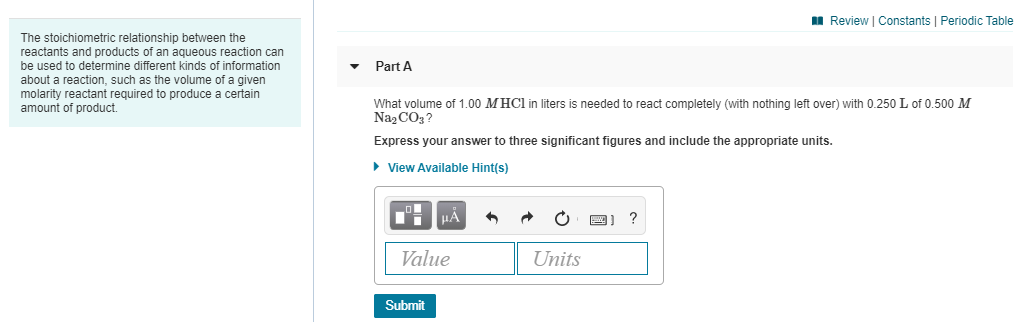Part A What volume of 1.00 M HCl in liters is needed to react completely (with nothing left over) with 0.250 L of 0.500 M Na2CO3? Express your answer to three significant figures and include the appropriate units. Part B A 419-mL sample of unknown HCl solution reacts completely with Na2CO3 to form 12.1 g CO2. What was the concentration of the HCl solution? Express your answer to three significant figures and include the appropriate units. A Review Constants Periodic...

• The stoichiometric relationship between the reactants and products of an aqueous reaction can be used to...

The stoichiometric relationship between the reactants and products of an aqueous reaction can be used to determine different kinds of information about a reaction, such as the volume of a given molarity reactant required to produce a certain amount of product. Hydrochloric acid (HCl) reacts with sodium carbonate (Na2CO3), forming sodium chloride (NaCl), water (H2O), and carbon dioxide (CO2). This equation is balanced as written: 2HCl(aq)+Na2CO3(aq)→2NaCl(aq)+H2O(l)+CO2(g) a. What volume of 2.50 M HCl in liters is needed to react completely...

• Part B What is the final volume in milliliters when 0.994 L of a 42.3 %...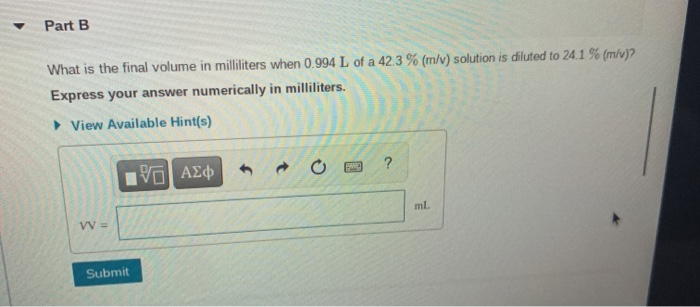Part B What is the final volume in milliliters when 0.994 L of a 42.3 % (m/v) solution is diluted to 24.1 % (m/v)? Express your answer numerically in milliliters. View Available Hint(s) ? Pa| ΑΣφ ml W = Submit 7 of 28 A Review Constants Periodic Table Part C A 894 ml NaCl solution is diluted to a volume of 1.02 L and a concentration of 6.00 M. What was the initial concentration? Express the molar concentration numerically. View...

• Part A What volume of 1.75 M HCl in liters is needed to react completely (with...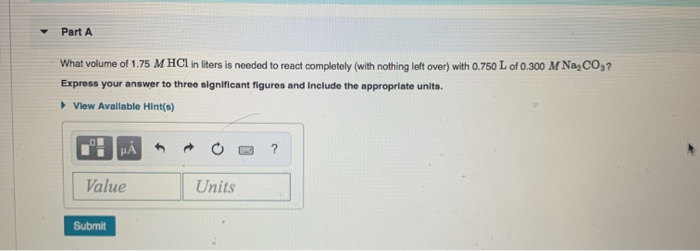Part A What volume of 1.75 M HCl in liters is needed to react completely (with nothing left over) with 0.750 L of 0.300 M NaCO3? Express your answer to three significant figures and include the appropriate units. View Available Hint(s) I HẢ * * 0 -3 ? Value Units Submit

• Determine the volume of 0.170 M NaOH solution required to neutralize each sample of hydrochloric acid....

Determine the volume of 0.170 M NaOH solution required to neutralize each sample of hydrochloric acid. The neutralization reaction is: NaOH(aq)+HCl(aq)→H2O(l)+NaCl(aq) 45 mL of a 0.050 M HCl solution Express your answer to two significant figures and include the appropriate units. 165 mL of a 0.885 M HCl solution Express your answer to three significant figures and include the appropriate units.

• Review I Constants 1 Periodic Table Part B What is the final volume V2 in milliliters...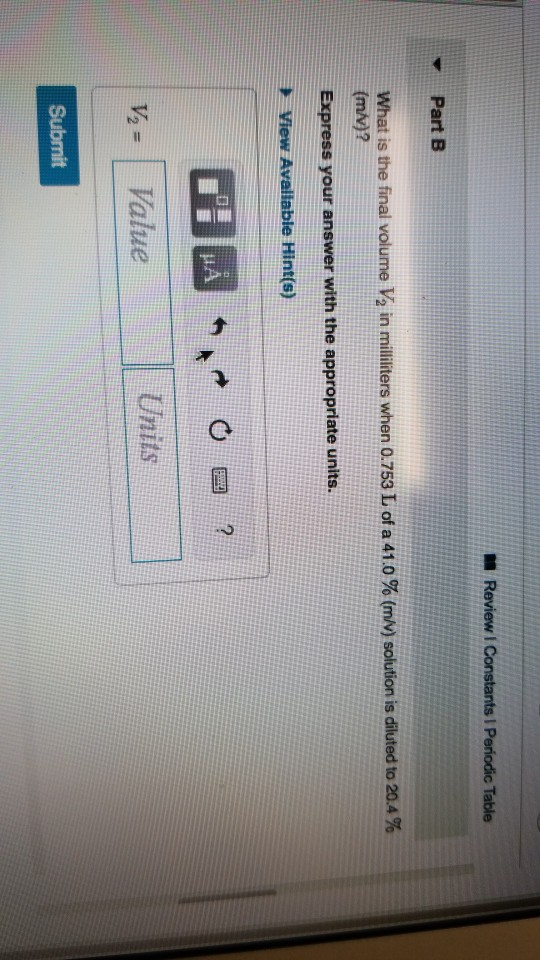Review I Constants 1 Periodic Table Part B What is the final volume V2 in milliliters when 0.753 L of a 41.0 % (m/v) solution is diluted to 20.4 % (m/v)? Express your answer with the appropriate units. View Available Hint(s) DA *MAN OR? Value Units V = Submit Review | Constants 1 Periodic Table Part C A 841 ml NaCl solution is diluted to a volume of 1.46 L and a concentration of 8.00 M. What was the initial...

• Solution Dilution a) What is the final volume in milliliters when 0.533 L of a 32.3 % (m/v) solution is diluted to 20.8...

Solution Dilution a) What is the final volume in milliliters when 0.533 L of a 32.3 % (m/v) solution is diluted to 20.8 % (m/v)? b) A 999 mL NaCl solution is diluted to a volume of 1.42 L and a concentration of 8.00 M . What was the initial concentration? How do I solve these? Thanks

• Review Constants Periodic Table Determine the volume of 0.155 M NaOH solution required to neutralize each...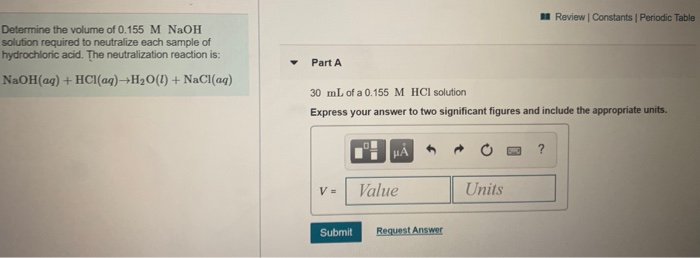Review Constants Periodic Table Determine the volume of 0.155 M NaOH solution required to neutralize each sample of hydrochloric acid. The neutralization reaction is: Part A NaOH(aq) + HCl(aq) +H2O(l) + NaCl(aq) 30 mL of a 0.155 M HCl solution Express your answer to two significant figures and include the appropriate units. 1: MÅ v - Value RO? Units Submit Request Answer Review Constants | Periodic Tab Determine the volume of 0.155 M NaOH solution required to neutralize each sample...

• A volume of 90.0 mL of a 0.750 M HNO3 solution is titrated with 0.730 M...

A volume of 90.0 mL of a 0.750 M HNO3 solution is titrated with 0.730 M KOH. Calculate the volume of KOH required to reach the equivalence point. Express your answer to three significant figures and include the appropriate units.